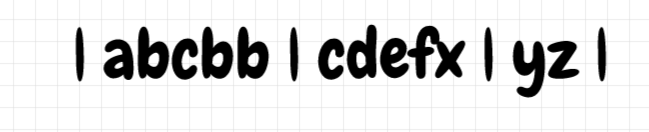Gaitonde's Revenge
Tag(s):

## Easy, Implementation, Strings, binary-search, epiphany8.0, medium

Problem
Editorial
Analytics

Gaitonde wants to take revenge against Sulaiman Isa. So he decides to send an army of characters to attack Isa. He has a team in the form of string $S$ of $N$ characters. He has to attack Isa on $Q$ days. Each of the $Q$$th$ day, Gaitonde has a string $T$, an integer $B$ and another integer $K$. He has to form string $T$ from string $S$ so that the order of characters in T must be same as that of S. However starting from the beginning, the characters in $S$ have divided themselves in groups of $B$ characters each except the last which has $\le B$ members.If there are remaining characters in the last, they will form their group. Since Gaitonde wishes to maintain diversity, he will select no more than K members from each group to form $T$. For each day, your task is to find whether it is possible to form $T$ from string $S$ as per rules, so that Gaitonde can attack Isa.

Input Format :-

• First line consists of single integer N, denoting the length of string S.
• Second line consists of string S of length N.
• Third line consists of single integer Q, denoting the total number of days Gaitonde will attack Isa.
• Each of the next Q lines consists of string of length M, an integer B, and another integer K. It means that for this query, the string S is divided into groups of B characters, and you are allowed to pick atmost K characters from each group of S so as to form T.
• All strings will only contain lowecase English letters.

Output Format :-

• Print Q lines: The ith line denotes the answer to ith day.
• Print "Yes" (without quotes) in new line, if it is possible to form string T from S as per rules, else print "No" (without quotes) in a new line.

Constraints :-

• $1 \le N \le 2*10^{5}$
• $1 \le Q \le 10^{5}$
• $1 \le \sum |T| \le 4*10^{5}$ , sum of length of T over all queries Q
• $1 \le B \le N$
• $1 \le K \le B$
SAMPLE INPUT
12
abcbbcdefxyz
6
abz 3 1
baz 3 2
abbby 3 2
abxc 5 2
acdxyz 5 2
SAMPLE OUTPUT
Yes
No
Yes
No
No
Yes
Explanation

For queries 1-4, the string is divided as shown.1) = abz and = 1, You can choose group 1 to form a, group 2 to form b and group 4 to form z. Note that we are choosing at max 1 character from each group.

2) = baz and = 2, It is not possible to form T.

Note, you can't choose first group to form ba and then last group to form z. Since, the order of characters in T must be same as order in string S.

3) = abbby and = 2. Choose group 1 to form a and b, group 2 to form b and b and group 4 to form y.

For queries 5-6, the string is divided as shown.5) = abxc and = 2, It is not possible to form T.

Note, you can form a and b from 1st group but xc can't be formed.  Since, the order of characters in T must be same as order in string S.

6) = acdxyz and = 2, choose group 1, 2 and 3 to form string T.

Time Limit: 1,0 sec(s) for each input file.
Memory Limit: 256 MB
Source Limit: 1024 KB
Marking Scheme: Marks are awarded when all the testcases pass.
Allowed Languages: Bash, C, C++, C++14, Clojure, C#, D, Erlang, F#, Go, Groovy, Haskell, Java, Java 8, JavaScript(Rhino), JavaScript(Node.js), Julia, Kotlin, Lisp, Lisp (SBCL), Lua, Objective-C, OCaml, Octave, Pascal, Perl, PHP, Python, Python 3, R(RScript), Racket, Ruby, Rust, Scala, Swift, Swift-4.1, TypeScript, Visual Basic

## CODE EDITOR

Initializing Code Editor...

## This Problem was Asked inChallenge Name

EPIPHANY 8.0

OTHER PROBLEMS OF THIS CHALLENGE
• Algorithms > String Algorithms
• Algorithms > Dynamic Programming
• Math > Combinatorics
Уведомления

?